Fit Analyses

# Weighted Analyses

If the errors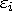do not have a common variance in the regression model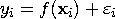a weighted analysis may be appropriate. The observation weights are the values of the Weight variable you specified.

In parametric regression, the linear model is given by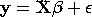Let W be an n×n diagonal matrix consisting of weights w1>0, w2>0, ..., and wn>0 for the observations, and let W1/2 be an n×n diagonal matrix with diagonal elements w11/2, w21/2, ..., and wn1/2.

The weighted fit analysis is equivalent to the usual (unweighted) fit analysis of the transformed model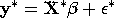where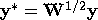,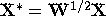, and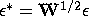.

The estimate ofis then given by

bw = (X'WX)-1 X'Wy

For nonparametric weighted regression, the minimizing criterion in spline estimation is given by
In kernel estimation, individual weights are
For generalized linear models, the function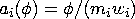for binomial distribution with mi trials in the ith observation,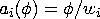for other distributions. The function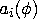is used to compute the likelihood function and the diagonal matrices Wo and We.

The individual deviance contribution di is obtained by multiplying the weight wi by the unweighted deviance contribution. The deviance is the sum of these weighted deviance contributions.

The Pearson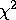statistic is
for binomial distribution with mi trials in the ith observation,
for other distributions.